[ Main page | Part I. Geometry and combinatorics | Part III. Multiplication | Part IV. Closures ]

# Coxeter groups Part II. Word processing

by Bill Casselman

### Introduction

 In working with Coxeter groups on a computer, the most basic questions to be answered are How to represent elements of the group? How to multiply two elements of a group? How to list elements of a group? There is probably no one best way to deal with these questions in all circumstances, but it will become apparent that I have a personal favorite, partly because it is theoretically the most interesting. In this Chapter we shall examine the first and second questions. Multiplication will be considered in Chapter III.

### Matrices

 In any Cartan realization, the elements of W are linear transformations, and one natural idea is to represent them by the corresponding matrices. In some circumstances, for example in low-dimensional graphics or investigating the geometry of regions of the Tits cone, this is a reasonable choice, but usually it is a very poor one. To avoid round-off errors (a potentially serious problem), it is a good idea to use a Cartan matrix with integral coordinates if it exists (as it does for the crystallographic groups), or the standard realization, in which case you are faced with arithmetic in cyclotomic fields. Storage (proportional to |S|2) is one problem, and the low speed involved in cyclotomic arithmetic is another. Also, testing inequalities involving cyclotomic numbers can be tricky. Why are round-off problems potentially serious? For finite and affine groups they probably aren't, but for groups where the Tits cone is acute it may well be - as a chamber wC appproaches its boundary in projective space, it becomes more and more difficult to separate two distinct chambers. This problem is not avoided with crystallographic groups, either, where integer overflow may occur. In practice, I have seen graphics programs have trouble with single precision calculations (about 7 decimal accuracy), producing images of chambers that were visibly awry near the Tits cone boundary.

### Vectors

A method that is subject to some of the criticisms in the previous section, but is nonethless sometimes convenient, is one which represents each element of W by a vector in |S| dimensions. First fix a vector u in the interior of the fundamental chamber C. Then an element w is characterized by either of its transforms wu or w-1u - in effect by the chamber wC itself, since no w will fix u. The vector is probably best expressed in terms of the coordinates wa = < a, w-1u > where a varies over the basic roots. The amount of storage involved is minimal, certainly. Then we have a very simple and efficient formula for reflection (where a = as):

 (ws)b = < b, (ws)-1u > = < b, s w-1u > = < b, w-1u > - < a, w-1u > < b, av > = wb - < b, av > wa

This is especially efficient because the number < b, av > (an entry in the Cartan matrix) will in practice be 0 most of the time and can then be ignored. This formula can be used to multiply two elements x and y if we know an expression y = s1 ... sn for y.

One nice feature of this representation of elements is that we can use it to find the reduced expressions to use in multiplication. We know that ws < w if and only if C and w-1C lie on opposite sides of the hyperplane a = 0 (a = as), that is to say if < a, Cw > = < w-1a, C > < 0, or in other words if wa < 0. So to find a reduced expression for w we let s be such that wa is negative and calculate ws. Repeat until there are no negative coordinates, when w = 1.

Exercise 1. (Research problem) Suppose the realization to be the standard one. Take u to be such that ua = 1 for all a. The coordinates of w-1u are cyclotomic. Is there a good way to tell whether (wu)a < 0 without using real approximation? To estimate carefully how good approximations have to be if you do use them? (This can certainly done for finite dihedral groups.)

### Words

 One natural way to express elements of W is in terms of one of its expressions as a product of generators. At first this seems a daunting idea, but in time it looks better and better. Its principal advantage is that there are certainly no problems with accuracy. Apparent disadvantages are the complexity of calculations - it is not at all clear where to begin - and possibly having to deal with words of arbitrarily large length. In particular, neither the problem of multiplication nor that of generation have immediately apparent solutions. These, at least, turn out not to be real difficulties, and for interesting mathematical reasons. As for the length of words, in practice it does not seem to be a problem except for elements where calculations are impractical for other, more serious, reasons. The first difficulty faced in this approach is to decide which of many, many possible reduced expressions by which to represent an element. There are two natural choices. The first is to single out what is called the ShortLex expression, which is lexicographically least (i.e. least in dictionary order) among all reduced expressions. This requires first ordering elements of S or, equivalently, indexing them. Consider the dihedral group of order 8 with generators s < t, for example. Every element except the longest one has a unique reduced expression, and the longest can be expressed as stst or tsts (according to the braid relation). The ShortLex expression for it is stst, since it comes before tsts in the dictionary. More precisely, the ShortLex expression for an element w has this recursive definition: (1) 1 corresponds to the empty word; (2) if s is the least generator such that sw < w then the ShortLex expression for w is s concatenated to that for sw. Sometimes it is convenient to use the InverseShortLex expression instead, which is the lexicographically least expression when read backwards. In this convention, the longest element above would be represented by tsts. For example, it is the InverseShortLex expression for w that we can find easily from the vector expression (wa), by choosing for length reduction the least a with wa < 0. In the rest of this lecture, we shall explore this purely combinatorial approach.

### Tits' word reduction

 The first interesting combinatorial result was found by Jacques Tits. In principle, in showing that one word in S is equivalent to another, it could be necessary to inflate the sizes of words as well as shrink them. Tits showed around 1968 that no inflation was necessary. Although this result will play no very practical role, the result is useful in hand calculation, and I will present here a slight modification of Tits' proof. Changing Tit's terminology slightly, I define reduction of a word in S to be the process of iterating these two transformations of words: deleting a pair s # s; replacing one side of a braid relation by the other. Here I use # to denote concatenation of words. Let R(w) be the set of all words obtained by all reductions of w. It is a finite set, although in general quite large. It includes, for example, all reduced expressions for the element represented by the word, which for even small groups can be huge. The longest element of H4, for example (a group of 14,400 elements whose longest element has length 60) has, Du Cloux tells me, 1,852,659,333,124,308 reduced expressions! At any rate, the number of such expressions for a long element can be reasonably expected to be huge. There are three properties of reductions that are straightforward: If y lies in R(x) then R(y) is contained in R(x); if y lies in R(x) and l(x) = l(y) then R(y) = R(x); R(s # x) contains s # R(x). Here, l(x) is the length of x as a word, not as an element of W. The third property is a special case of the more general fact that reduction is context-free: that R(x # y # z) contains x # R(y) # z. Theorem 1. (Jacques Tits) In order for two words x and y to be equivalent, it is necessary and sufficient that their reduction sets R(x) and R(y) have an element in common. Proof. If the intersection is non-empty, then certainly the two are equivalent. The non-trivial half of the Theorem is to show that if x and y are equivalent then R(x) and R(y) intersect. We may assume that l(y)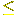l(x). Now, the points (i, j) with ji look like this in the plane: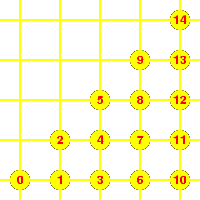In particular, as the illustration shows, there is a natural order in which to count such points, and the proof proceeds by induction on the index of the pair l(x), l(y). If l(x) = 0 the assertion is trivial. Suppose now that l(x) > 0, that l(y)l(x). Our induction assumption is that the Theorem is true for all pairs x*, y* with l(x*) < l(x) or l(x*) = l(x) but l(y*) < l(y). Suppose that there exists z with l(z) < l(x) lying in R(x). Of course z and y are equivalent. If l(y) < l(x) than (z, y) < (y, x) since l(y) < l(x). If l(y) = l(x) then (z, y) < (y, x) since l(y) = l(x) but l(z) < l(y). Either way, we deduce by induction that R(z) and R(x) overlap, hence R(x) and R(y) as well. So we may assume from now on that all elements of R(x) have the same length as x. Since l(x) > 0, x = s # x* for some s. If R(x*) contains elements of length less than that of x*, then since R(x) contains s # R(x*) so does R(x) contain elements of length less than that of x, a contradiction. So we know that all elements of R(x*) have the same length as x*, which is l(x) - 1. Suppose that y = s # y*. Then x*, y* satisfy the induction hypothesis, so that R(x*) and R(y*) have an element in common. But then so do s # R(x*), s # R(y*), hence R(x) and R(y). So now we may assume that y = t # y* with t different from s. In these circumstances, l(y) must be the same as l(x). For suppose that l(y) < l(x). The elements s # y and x* are equivalent. Therefore by the induction assumption R(s # y) and R(x*) have a common element. But since all elements in R(x*) have the same length, l(s # y) = l(x*) = l(x) - 1. And R(s # y) = R(x*). Therefore s # sy lies in R(x), and y lies in R(x). This contradicts our current assumptions. So now we may assume (a) l(y) = l(x), and (b) x = s # x*, y = t # y*, with s and t distinct. Since x and y are equivalent, as elements in W they satisfy sx < x, tx < x, sy < y, ty < y. Therefore they are longest in their common Ws, t-coset. Hence there exists a distinguished z in Ws, t with x, y equivalent to ss, t z, where sz > z, tz > z, ss, t the longest element in Ws, t. Then y* is equivalent to s # t ... z, x* is equivalent to t # s ... z, and by induction R(y*) = R(s # t ... z), R(x*) = R(t # s ... z), and R(x) = R(s # x*) = R(s # ts ... z), R(y) = R(t # y*) = R(t # st ... z). But the last two are equivalent according to the braid relation, hence R(x) = R(y). QED Because of the potentially huge size of R(x), it is definitely not usually practical to calculate it. Nonetheless, Tits' reductions are easy enough to carry out by hand in simple cases, and his Theorem assures that this effort will not be fruitless. Also, in principle Tits' algorithm tells us how to calculate products. For this, choose an ordering of the elements of S (or, equivalently, index S). Assign to each element in W its unique ShortLex word. Calculating the product of two words x and y means finding the ShortLex expression of the concatenation z of the words. We can do this by calculating R(z) and listing in order in order all those words in it of shortest length. There are standard computer algorithms to do all these operations fairly efficiently, given the essential inefficiency of the process itself. Exercise 2. (A minor research problem) Turn this proof into an algorithm that starts with a chain of relations allowing either insertions or deletions and winds up with a pair of reductions.

### Finite automata and regular expressions

 We are going to be interested in recognizing patterns in reduced expressions for elements of W. The simplest patterns of importance will turn out to be regular expressions, those which can be recognized in a particularly simple fashion. Let's look again at the dihedral group of order 8. Here is a list of all the ShortLex expressions of its elements: 1, s, t, st, ts, sts, tst, stst. This can also be described by a diagram: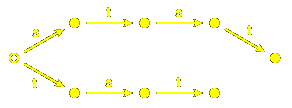in which paths starting at the dotted disc parametrize ShortLex words in the group, matching labeled edges between nodes and generators in a word. No surprises here; there are exactly as many nodes in the diagram as there are elements in the group. Something slightly more curious is that paths in this smaller diagram also parametrize the same words: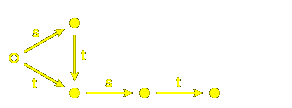But the real point to be made occurs with the infinite group A1~. The words representing elements can be represented by paths in this diagram, where again elements are in bijection with nodes: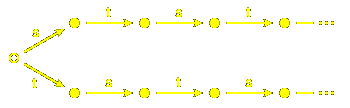but also by paths in this one: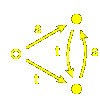Of course, this is a wonderul compression! It is the first example we have seen of a phenomenon that we shall see much of later on, and one that seems to be ubiquitous in this subject: infinite Coxeter groups are often represented well by finite structures. Diagrams of the sort we are looking at - roughly speaking, directed labeled graphs together with a designated starting point - are called automata or state machines in the theory of languages. They play an important role in how computers parse input, among other things. The sets of strings that are recognized by such diagrams are called regular expressions. They are called automata because paths tracing through one follow a program with a strictly limited number of options - if they have arrived at a node, then what they can do subsequently depends only on that node, not on the history of how they got to it. The paths have limited memory, like a simple mechanical toy. Exercise 3. If S is any set, the set of all words in S is a regular language commonly expressed as S*. If S = {s, t}, design a finite automaton that recognizes S*. (Edges are allowed to go from a node to itself.)

I believe, for reasons that will be laid out later on, that regular expressions will prove to be unavoidable in understanding important phenomena associated to Coxeter groups. How important remains to be seen. Nonetheless, for this reason it seems useful to look at them in more detail. There are many points that haven't been touched upon yet, and a few that haven't been made very precise.

I should also point out that by no means all interesting languages are regular. The simplest example is that of balanced left and right parenthetical expressions. The point is that a regular language has only a limited memory, whereas whether or not a parenthesis `)' is balanced somewhere in an expression by a `(' may involve searching back to the beginning of the expression.

#### Deterministic automata

The following are examples of how one normally writes positive rational numbers:

13, 2/4, 20/47, 1/24.

These are not:

13/1, 02/4, 0/0 .

Note that we are not restricting ourselves to reduced fractions. We can put together a recipe for expressing a positive rational number: (1) To write a positive integer we write a sequence of non-negative digits, starting with a positive one. (2) To write a positive rational number, write a positive integer, followed optionally by a slash / followed by a positive integer other than 1. This recipe is not trivial to read, and for most people it is easier to understand if we encode it in a diagram: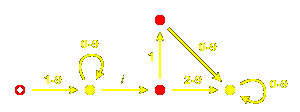This looks like a finite automaton, but there is a new feature - the red nodes. The point is that not all paths in this diagram lead to an acceptable expression for a fraction. The prefix of a valid expression for a fraction may not be itself valid. For example, the empty string is not acceptable. Also, a single 1 in the denominator is not acceptable. In this diagram, only those paths leading to a yellow node correspond to acceptable expressions. The yellow nodes are said to be the accepting states of the automaton.

So we have a precise definition: a deterministic automaton is a directed graph together with an alphabet (also called the set of imput symbols). The edges in the graph are labeled by symbols from the alphabet, and there is at most one edge with a given label leaving any node. Some of the nodes are designated as accepting states and one of them as the start state. To such a graph is associated a language in the alphabet S, namely those words accepted by a path in the automaton. A path is a sequence of node n0, n1, ... , nm and it accepts the word s1 ... sm if (a) n0 is the start state; (b) nm is an accepting state; (c) there is an edge from nk-1 to nk labeled by sk. If the graph is finite, the language is called regular. This is a crucial restriction, since for any language we can make a recognizing automaton from the tree of all words in the language, which I call the tautologous automaton of the language.

Exercise 4. Design a finite automaton that represents real numbers.

Why can't we expect a finite automaton to recognize reduced fractions? Whether an expression is recognized by a finite automaton depends on its form, not its content - its syntax, not its semantics. Deciding whether a fraction is reduced or not involves thinking about the meaning of fractions, not just their form. We shouldn't expect anything as simple as a finite automaton to handle this sort of thing. Syntactical analysis is often, however, a preliminary to semantic interpretation.

#### Non-deterministic automata

There are also non-deterministic automata. Or, more properly, not-necessarily-deterministic automata. I abbreviate the term as NDA. There are three modifications: (1) There may be several starting states; (2) there may be unlabeled edges; (3) there may be several edges with a given label leaving a node. If s1 ... sm is a word in the alphabet, a path accepting this string is a sequence of nodes n0, n1, ... , nm in the automaton such that (a) n0 is one of the start states; (b) nm is one of the accepting states; (c) from nk-1 to nk there is a directed sequence of edges, one labeled by sk and the rest unlabeled. What is new is that the path is not determined by the word; there may be several paths all accepting the same word. Such diagrams are technically very useful, but do not actually enlarge the meaning of the term `regular expression'. Equivalent automata are those that recognize the same words in the same alphabet.

Proposition 2. Every finite NDA is equivalent to a finite deterministic one.

Proof. Suppose given an automaton A. How is an equivalent deterministic one constructed? First of all, nodes in the new automaton are going to be defined as certain subsets of nodes in the original, which I'll call A. And there are two simple auxiliary constructions to be applied in the course of the construction. (a) If X is any set of nodes in A, define its saturation S(X) to be the union of X and all nodes reachable from X by an unlabeled edge. A set is called saturated if it is its own saturation. (b) If X is a saturated set of nodes in A and s an input symbol, define X # s to be the saturation of the set of nodes which are the target of an edge labeled by s leading from a node in X. It is entirely possible that X # s = X # t for distinct s and t.

The nodes of the new automaton are the saturated subsets of the nodes of A. There is an edge labeled by s from X to X # s. The start state is the saturation of the set of start states of A. A node is accepting if it contains an accepting node from A. I leave it as an exercise to verify that this equivlent to A.

The new automaton may be huge. In practice, most of the new nodes may be thrown away, because they are not attainable from the start state. As an extreme example, if A is itself deterministic then the only attainable nodes are the singletons. The standard algorithm for constructing the new automaton constructs only those new nodes which are attainable.

The potential exponential growth does occasionally happen.

#### Irredundant automata

A node in an automaton is called redundant if no path from a start state reaches it. Eliminating these produces an equivalent automaton.

An automaton is called complete if for each node n and each input symbol s there exists an edge from n labeled by s. Any automaton A can be converted easily into an equivalent complete one by adding to it a dead state. This new node is not accepting, and all edges from it lead to itself. If n is a node of A without an edge from it labeled by s, then in the new automaton there will be an edge labeled by s leading to the dead state.

#### Inversion

The usefulness of non-deterministic automata becomes clear when one tries to build an automaton recognizing the same strings as an automaton A, but read backwards. We simply define a new automaton to have as its nodes the same nodes of A. Its starting states are the accepting states of A, the single accepting state of the new automaton is the start state of A, and edges in the new automaton are reversed from those of A. That is to say, to an edge from x to y in the original corresponds an edge from y to x in the new one, with the same label. This is a very simple construction, but almost always the new automaton will be non-deterministic. Thus:

Proposition 3. The inverted words of a regular language also form a regular language.

This accounts for the result that InverseShortLex is a regular language if ShortLex is.

#### Concatenation

If X and Y are languages, then the concatenation of the two is the set of all words x # y constructed by concatenating a word x from X with on from Y. It is denoted X # Y.

Proposition 4. The concatenation of two regular languages is again regular.

Proof. Let X and Y be the two languages, A and B recognizing automata. We want to construct an automaton recognizing X # Y in simple terms from A and B. An example will motivate the construction, which might otherwise seem mysterious. Suppose X is the language containing just the words a and ab, while Y is that containing just b and bc. Then X # Y is made up of ab, abb, abc, abbc. The minimal deterministic automata for these three languages are

 X: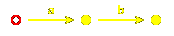Y: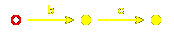X # Y:The problem is that there is no way to see how the automata for X and Y are related to that for X # Y. The source of the difficulty is that the b in abb is different from that in abc - one comes from X and the other from Y. There is apparently no direct way to build a deterministic automaton directly from the two components, but there is nonetheless a simple solution: instead of trying to build a deterministic automaton recognizing X # Y we shall build a non-deterministic one. The dual source of the letter b will be dealt with (at least implicitly) by allowing two edges from a given source labeled by b. The actual construction for an arbitrary X and Y is this:

Make up a new version of A by adding to a copy of it a new terminal node z, with unlabeled transitions to it from each accepting state in A. Now add on to this in turn a copy of B, with unlabeled transitions from z to any of the starting states of B (only one, of course, if B happens to be deterministic). The accepting nodes of the new automaton are just the accepting states of B.

#### Repetition

If X is a languages, then the repetition of X is the language of all words obtained by concatenating 0 or more words from X. It is denoted X*.

Proposition 5. The repetition of a regular language is again regular.

Proof. Suppose A recognizes X. Add two nodes to a copy of A, at the beginning and end. From the first add unlabeled edges to all start states of A, and from each of the accepting states add an unlabeled edge to the last. From the last back to the first also add an unlabeled edge. Make each of the new nodes accepting, as well as those of A. Add the first to the start states.

#### Alternation

If X and Y are languages, then the alternation of the two is the set of all words w which lie in either X or Y. It is denoted X | Y.

Proposition 6. The alternation of two regular languages is again regular.

Proof. Suppose X, Y, A, B as above. Make up a new automaton by starting with copies of A and B. Add in a single new node, with unlabeled edges leading from it to all start states of either A or B. Make it the single start state of the new automaton.

#### Negation

If X is a regular language, then the words in the same alphabet which are not in E also make up a regular language. This new language is called the negation of the original language X and is denoted !X. Keep in mind that this negation is with respect to the given alphabet.

Proposition 7. The negation of a regular language is regular.

Proof. Suppose that A is a complete automaton recognizing X. The recognizing automaton for its negation has the same nodes, edges, and start state as A, but its accepting states are the complement of the accepting states in A.

#### Intersection

If X and Y are languages, then the intersection of the two is the set of all words simultaeously in both languages. It is denoted X & Y.

Proposition 8. The intersection of two regular languages is again regular.

Proof. It is the negation of the alternation of their negations.

#### Minimal automata

Any language is recognized by a unique smallest automaton. There is an efficient algorithm to compute the equivalent minimal automaton from any given finite one.

Here's an example we have seen already. The tautologous automaton recognizing reduced words for A1~ isBut now some of the nodes are equivalent in the sense that the paths out of these nodes are the same - i.e. the options for continuing a word from that node are the same. In this picture, all the nodes coloured green are equivalent since all paths out of any of them is just a repeated st ... , and all those coloured blue are also equivalent: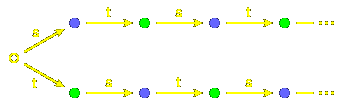We can collapse all of the same colour to a single point, to get a new equivalent automaton: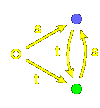This construction is always valid, but a bit of care is required. It is not the full set of words leaving a node which is important, but just those which lead to acceptable nodes. For example, a few extra edges could always be added to a dead state without changing things substantially. If A is any automaton, define two nodes n1 and n2 to be equivalent if they have the following property: a word w leads from n1 to an accepting state in A if and only if it leads from n2 to an accepting state. Define a new automaton whose nodes are the equivalence classes of nodes in A. It start state is the class containing the start state of A. Its accepting states are those classes containing accepting nodes of A. There is an edge labeled s from a class N1 to a class N2 if there is an edge from n1 in N1 to n2 in N2 labeled by s. I leave it as an exercise to verify that this is an equivalent deterministic automaton.

#### The minimization algorithm

There is an algorithm for producing the minimal automaton equivalent to a given one which is remarkably efficient. To explain it, I begin by putting the problem more constructively. Let A be the given automaton, which for the moment I assume complete. Nodes n1 and n2 of A are called equivalent if for every word w in the alphabet of A the nodes n1 # w and n2 # w are either both accepting or both not. Break this down into more a more explicit test. Divide the nodes into two blocks, the accepting nodes and the rest. Call two nodes equivalent of order 0 if they are both in the same block. Inductively, call node n1 and n2 equivalent of order m+1 if they are equivalent of order m and the nodes n1 # s and n2 # s are also equivalent of order m for all symbols s. Thus they are equivalent if they are equivalent of all orders.

Suppose we have already found the blocks B of elements equivalent of order m, and suppose there are k input symbols. Then we partition each block B into smaller blocks BC1, ... , Ck, where BC1, ... , Ck is the set of b in B such that b # si lies in the block Ci, for each i = 1, ... , k. If there are N blocks of order m equivalence, then a priori there might be as many as Nk+1 blocks of order m+1, a potentially disastrous super-exponential growth.

In fact there exists an algorithm with time dependence O(k n log n) if n is the number of states in the original automaton. This works because most of the blocks BC1, ... , Ck will be empty, so the idea is to find away of going from one order to the next by somehow automatically excluding the empty ones.

This algorithm solves a slightly more general problem, one which occurs when dealing with Coxeter groups. Suppose we are given a collection of maps fi, i varying over a set S, and a partition of a set X into blocks Xi. Two elements x and y are called equivalent if any iteration of the f's applied to them finds them in the same block. We shall call an element dead if all the f's take it to itself. It works like this, in a rough description:
 Start with the partition into two blocks, accepting and non-accepting. Maintain a list of pairs (B, s), to be processed in the order in which they are put on the list, and start the list off by putting all (B, s) on the list where B is the block of accepting states and s ranges over all the input symbols. While this list is not empty: take a pair (B, s) off the list. For each node y in B look at all nodes x with x # s = y. Record the block Bx in which x appears. After all y have been examined, look at each of the blocks B* that have been met in this way. If the total number of times they have been encountered is not equal to the total number of elements in B*, then divide B* into two parts, the x such that x # s lies in B#, and the rest. Let C be the smaller of these two subsets of B*. Put (C, s) on the process list for all input symbols s. Loop. In implementing this, before beginning make for each y and s a list of all nodes x with x # s = y. As we process the elements y of a block B we maintain a list of all x we meet with x # s in B as well as the block to which x belongs. We also maintain a list of those Bx, but each one listed only once. After we have examinedd all y in B, we go through the list of the B* met, comparing it to the set of x in it. If we do create a new block C by removing elements from B* we do one of two things: put (C, s) on the process list if (B*, s) is on that list; or if not then we put the smaller of (C, s) and (B*, s) on the list.

### Automata and Coxeter groups

 Let's look at the ShortLex words for a more complicated infinite Coxeter group, that with Coxeter diagram. It is an affine group, and we shall look at it as generated by affine reflections. There are three generators, which I write as 1, 2, 3. Each element of the group is represented by a ShortLex word, hence a path of coloured arrows from the chamber C to the chamber wC. Because of uniqueness, the paths all together make up a tree with C as its root.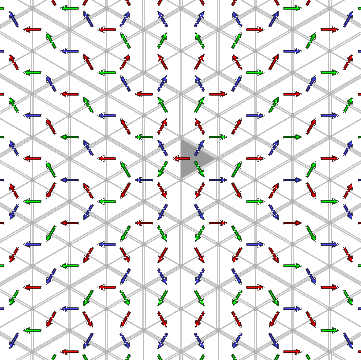Certain things are not difficult to see in this image. Every non-empty ShortLex expression starts with one of the three symbols 1, 2, or 3, so the chambers other than C are partitioned into three corresponding regions of the plane. Because of the ordering of the three, (1) the w starting with 1 are those chambers on the side of the line of reflection a1 = 0 opposite to C; (2) those w starting with 2 are those chambers on the side of the line of reflection a2 = 0 opposite to C, but not already in the first region; (3) those starting with 3 are those chambers on the side of the line of reflection a3 = 0 opposite to C, but not already in either of the previous two.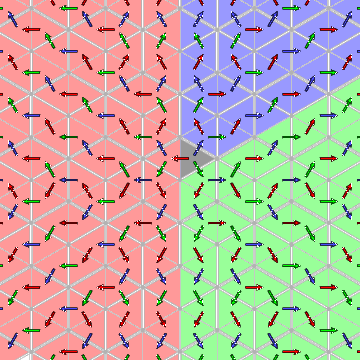It is significant that each of these regions is convex. Indeed, it appears that all of the regions comprised of a single chamber together with its successors in the tree are convex. We shall why this is so in a moment. The question is whether we can recognize in this tree a repetition of patterns similar to that in the tree for A1~. The answer is that we can. For example, there exist some simple cycles 132 ...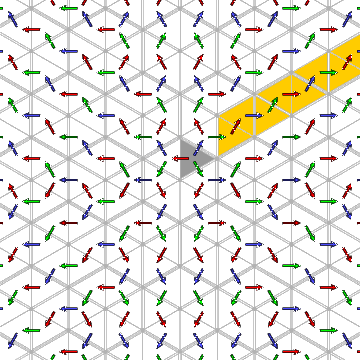There are lots of such cycles - in the following picture, each yellow chamber represents the same position in such a cycle:But there are more interesting repetitions: Start out at C and move by 3. You arrive at essentially the same pattern if you follow this by 1213. What do I mean by `the same pattern'? That the tree of paths leading out of the two chambers are congruent. If you pick up one of these trees, so to speak, and shift it rigidly to the other chamber, you can then lay it down upon the tree leading out of the second. (Of course I am not claiming to prove this, but all evidence is in favour of the assertion.) There are other chambers equivalent to these two, too. All such positions are shown here: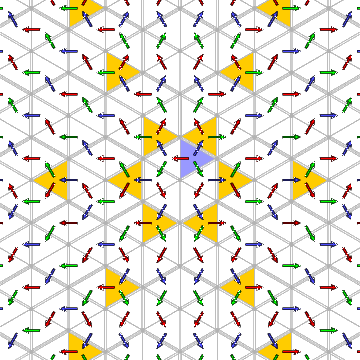Two chambers will be called ShortLex congruent if the pattern of transitions in the ShortLex tree out of each of them is the same. If this happens, then there exists an element of the Weyl group transforming one of these patterns onto the other. It turns out for A2~ as for A1~ (although we have only suggested that it is true, certainly not proven it) that the set of all ShortLex expressions for this group is a regular language, or in other words that there are a finite number of congruence classes. Here is the corresponding finite automaton: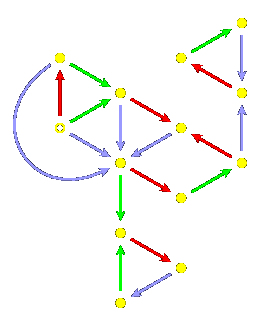Note that there are indeed loops 132 and 1213, as well as 123. This is not an isolated case. It is a very beautiful result of Brigitte Brink and Bob Howlett that: Theorem 9. For any Coxeter group, there are finite automata recognizing ShortLex expressions, InverseShortLex expressions, and reduced words in the group. We shall look at the proof in subsequent sections. That InverseShortLex is a regular language follows formally from the regularity of ShortLex, since it is just the reversed language. It is reasonable to think of both of these as lacking in significance, perhaps to be considered results in applied rather than pure mathematics. But that reduced words should also be recognized by a finite automaton must be a fundamental property of Coxeter groups. For finite and affine groups it is not a very deep result; it is equivalent to other more familiar properties of these groups. But for other groups it is not at all a trivial fact, and that it has taken so long to be discovered is likely just a sign that not much work has been devoted to these groups. Here is the finite automaton recognizing reduced words for A2~: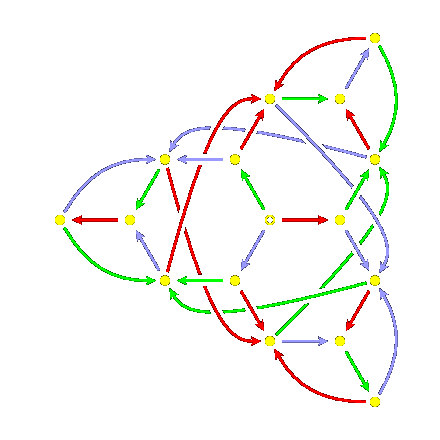Its symmetry arises from the symmetry of the Coxeter diagram. Embedded in it are three hexagons, which are the finite automata for the dihedral groups of two generators, each of order 6. The minimal finite automaton recognizing reduced words for a finite Coxeter group is always the same size as the group itself - this is essentially because all words terminate in the longest element. Exercise 5. Prove this last assertion - that any finite automaton recognizing the reduced expressions for a finite Coxeter group have to have size at least that of the group itself.

### Minimal roots

The key to the proof of the Theorem of Brink and Howlett is a more fundamental result of theirs about the roots of a Cartan realization of a Coxeter group. One positive root b is said to dominate another positive root a if the two roots are distinct and the intersection of the half-space a > 0 with the Tits cone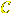is entirely contained in the half-space b > 0. In other words, if whenever wC lies in the region a > 0 then it also lies in the region b > 0, equivalently if whenever w-1a > 0 then w-1b > 0 also. I call a root minimal if it does not dominate any roots. (This notion was first applied seriously by Bob Howlett and Brigitte Brink. Their terminology differs from mine - In their joint paper they use the term elementary, and Howlett still prefers this. In a subsequent paper Brink uses the term dominance minimal, to take account of the distinction between the partial ordering on roots determined by dominance and another one to be introduced later on.) For finite groups, the Tits cone is all of V, so there is no dominance and all positive roots are minimal. For affine groups the concept is also simple. In this case the positive roots are of the form a + c for all positive roots a of the associated finite root system and non-negative integer constants c, as well as -a + c where a is positive and c is a positive integer. Thus whenever one root dominates another the root hyperplanes must be parallel. The basic ones are the basic roots of the finite system together with -a + 1, where a is what is common called the dominant root (although there is no relation with the dominance defined here). The minimal roots are those of the form a or -a + 1 where a is positive in the finite system. But for other groups the concept is more subtle. Here is what happens for the group with Coxeter diagram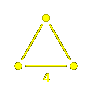: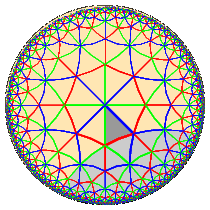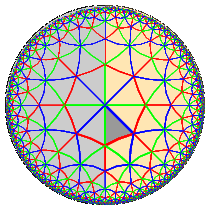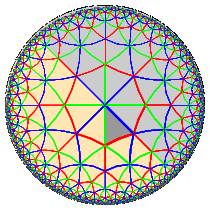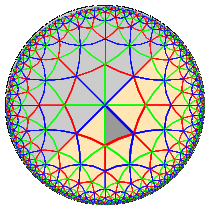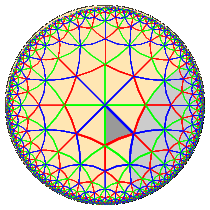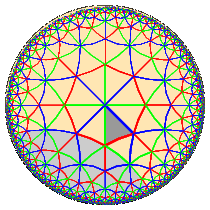In this and a few other simple cases the minimal roots all touch the chamber C, but that does not always happen.

The fundamental result of Brink and Howlett , upon which all their non-trivial results depend, is that:

Theorem 10. For any Coxeter group there are only a finite number of minimal roots.

Bob Howlett remarks somewhere that he thinks this is a fundamental fact about Coxeter groups, even though there seem to be few applications of it to other properties, and I agree with him.

I am not going to prove the theorem of Brink and Howlett here, but I shall explain some more elementary properties of minmal roots.

There are two interesting order relations among positive roots. One is dominance, which we have seen already. The other is more closely related to how the positive roots are generated. Every positive root is equal to the transform of a basic root by and element of W. Define the depth of a root p to be the least length of a w such that w-1 p < 0. This is also 1 less than the least length of a w such that w-1 p is a basic root. Geometrically, it is the length of the least gallery starting at C with terminal chamber in the region p < 0. Thus the depth of a basic root is 1. Define pq if q = w p, where the depth of q is equal to the sum of the depth of p and the length of w. We thus have a labeled directed graph: there is an edge from p to q labeled by s in S if d(q) = d(p) + 1 and s p = q, and pq if there exists a sequence of edges in this root graph from p to q.

1. The minimal roots are minimal in this order. 2. If p is a minimal root and s in S, then exactly one of these holds: (a) p = as, and s p < 0; (b) s p is another minimal root; (c) s p dominates as. Thus we get the minimal root reflection table. In cases (a) we write s p = -, in case (c) we write s p = +, else s p = q. The minimal root reflection table is an important tool in algorithms involving Coxeter groups.

The root hyperplanes p = 0 and q = 0 intersect inif and only if the group generated by sp and sq is contained in the conjugate of some finite dihedral group Ws, t. The product st will thus be of order dividing ms, t.

Lemma. If p dominates q and wq is a positive root then wp is also a positive root and wp dominates wq.

If p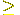q and p is minimal then so is q. If p dominates q then thedepth of p is greater than or equal to to that of q.

Brink's thesis.

Coxeter distance. Different definitions of depth. Structure of the root graph?

### Building the automaton

 For each w in W let w be the SL continuation, the set of all x in W such that the ShortLexword for w is a prefix of that for x. Then w # s lies in ShortLex if and only if ws lies in Cw and then Cws = Cw cap w Cs. The region Sw = w-1Cw is the ine intersection of minimal root half-spaces. Use the equivalent: w # s is in ShortLex if and only if s lies in Sw and then Sws = s Sw cap Ss. (consistency: C at least lies in this intersection since C is contained in s Sw). Distinguish geometry from elements. Proof: uses that if r is a minimal root then either (1) s r is again a minimal root; or (2) r = as and s r < 0 or (3) s r dominates as. The Sw determine the minimal automaton recognizing ShortLex or reduced words. For the second, need to know Ss = { as } while for the second { as } union s at (t < s) One advantage of knowing the automaton for ShortLex is that generation of elements is then easy.

### Other occurrences of regular expressions in Coxeter groups

Proposition 11. The reduced words for those elements of W with a unique reduced expression form a regular language.

Proof. The reduced words are a regular language, and those which uniquely represent an element are, by Tits' Theorem, those which do not contain either side of a braid relation. But those words in S which contain any one side of a particular braid relation form a regular language, hence so do those containing any side of a braid relation, since alternation preserves regularity. But the complement of a regular language is regular, and the intersection of two regular languages is regular.QED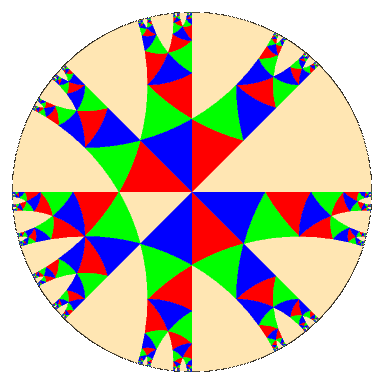The coloured chambers are those representing elements with unique expressions.

### References

 James Humphreys, Reflection groups and Coxeter groups, Cambridge Press, 1990. B. Brink, The set of dominance-minimal roots, preprint, University of Sydney, 1994. Available at http://. This is very different from the article with the same name published in the Journal of Algebra. It is in some ways more readable. B. Brink, The set of dominance-minimal roots, Journal of Algebra 206 (1998), pp. 371-412. B. Brink and R. Howlett, A finiteness property and an automatic structure for Coxeter groups, Math. Ann. 296 (1993), pp.179-190. W. Casselman, Automata, informal lecture notes (in PostScript format), 2002. W. Casselman, Automata to perform basic calculations in Coxeter groups, in Representations of groups, CMS Conference Proceedings 16, A.M.S., 1994. W. Casselman, Multiplication in Coxeter groups, to appear in the Electronic Journal of Combinatorics. A. Aho and J. Ullmann, Design and analysis of computer algorithms, Addison-Wesley, 1974. Fokko du Cloux, Un algorithme de forme normale pour les groupes de Coxeter, preprint, Centre de Mathématiques à l'École Polytechnique, 1990. Fokko du Cloux, A transducer approach to Coxeter groups, J. Symb. Computation 27 (1999), pp. 311-324. J. Tits, Le problème des mots dans les groupes de Coxeter, Symposia Math.1 (1968), pp. 175-185.